# Qlik Design Blog

All about product and Qlik solutions: scripting, data modeling, visual design, extensions, best practices, etc.Employee

## Recipe for a Pareto Analysis

“Which products contribute to the first 80% of our turnover?”

This type of question is common in all types of business intelligence. I say “type of question” since it appears in many different forms: Sometimes it concerns products, but it can just as well concern customers, suppliers or sales people. It can really be any dimension. Further, here the question was about turnover, but it can just as well be number of support cases, or number of defect deliveries, etc. It can in principle be any additive measure.

It is called Pareto analysis. Sometimes also known as 80/20 analysis or ABC analysis.

The logic is that you first sort the products according to size, then accumulate the numbers, and finally calculate the accumulated measure as a percentage of the total. The products contributing to the first 80% are your best products; your “A” products. The next 10% are your “B” products, and the last 10% are your “C” products.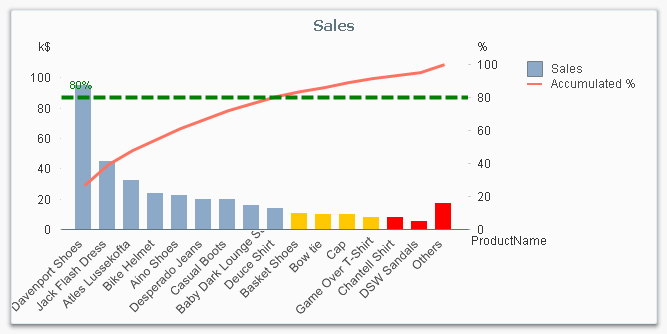And here’s how you do it in QlikView:

1. Create a pivot table and choose your dimension and your basic measure. In my example, I use Product and Sum(Sales).

2. Sort the chart descending by using the measure Sum(Sales) as sort expression. It is not enough just to check “Sort by Y-value”.

3. Add a second expression to calculate the accumulated sales value:
RangeSum(Above(Sum(Sales), 0, RowNo()))
Call this expression Accumulated Sales. The Above() function will return an array of values – all above values in the chart – and the RangeSum() function will sum these numbers.

4. Create a third expression from the previous one; one that calculates the accumulated sales in percent:
RangeSum(Above(Sum(Sales), 0, RowNo())) / Sum(total Sales)
Format it as a percentage and call it Inclusive Percentage.

5. Create a fourth expression from the previous one; one that calculates the accumulated sales in percent, but this time excluding the current row:
RangeSum(Above(Sum(Sales), 1, RowNo())) / Sum(total Sales)
Format it as a percentage and call it Exclusive Percentage.

6. Create a fifth expression for the ABC classification:
If([Exclusive Percentage] <= 0.8, 'A', If([Exclusive Percentage] <= 0.9, 'B', 'C'))
Call this expression Pareto Class. The reason why the Exclusive Percentage is used, is that the classification should be determined by the lower bound of a product’s segment, not the upper.

7. Create a conditional background color, e.g.
If([Pareto Class] = 'C', LightRed(), If([Pareto Class] = 'B', Yellow()))

You should now have a table similar to the following. In it you can clearly see the classification of different products.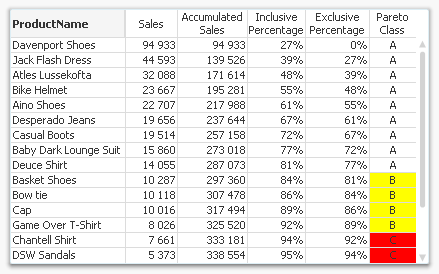In this table, there are five different expressions that you can use for Pareto analysis. The graph in the beginning of this post uses Sales and Inclusive Percentage for the bars and the line, respectively; and Pareto Class for the coloring of the bars.

Further, you may want to combine the Pareto Class and the Exclusive Percentage into one expression:

Pareto Class =
If(RangeSum(Above(Sum(Sales),1,RowNo())) / Sum(total Sales) <= 0.8, 'A',
If(RangeSum(Above(Sum(Sales),1,RowNo())) / Sum(total Sales) <= 0.9, 'B', 'C'))

Good luck in creating your Pareto chart.

HIC

Further reading related to this topic:

Recipe for an ABC Analysis

Recipe for a Pareto Analysis – RevisitedNot applicable

I'm also looking for a solution such as Rameen. Based on the 80-20-Analysis I want to know what number of products/customers etc. belong to Category A, B and C. Any suggestions/solutions?

236 ViewsPartner

thanks very much!

236 Views
Valued Contributor

Henric, thanks for the information.

236 Views
Contributor

Its great!

236 ViewsPartner

very good example!

Great236 ViewsNot applicable

Thanks a lot for posting such a useful tip. I tried every thing and succeded but i am unable to set the conditional background colour. Could you please let me know where exactly i need to write the cnditional background color for A. B and C values?

236 ViewsEmployee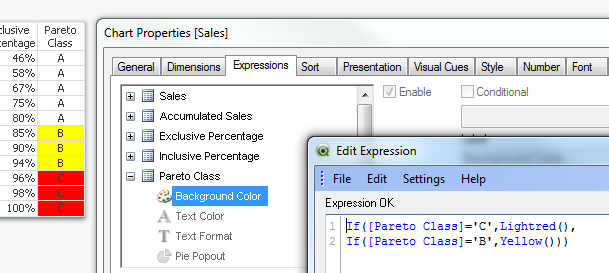236 ViewsNot applicable

Thanks a lot for the quick reply 🙂

236 ViewsLuminary

Hi,

I´m currently trying to copy this but I´m unable to adjust my formula:

I´m using a variable called vCalcTotalYear1 to usually calculate my sums.

Therefore I´m struggling with point number three. I tried to adjust my formula as follows:

RangeSum(Above(=\$(=vCalcTotalYear1)), 0, RowNo()))

My question is maybe not just related to the pareto recipe. But I thought you could help me the best as you know what I´m trying to do. I appreciate very much that you shared this document with the community. This makes easy for new users to get started quickly with smart analysis.

Best regards,

Carolin

236 ViewsEmployee

It depends on what you have in the variable. If it contains the text string 'Sum(Sales)' or similar, then you should use

RangeSum(Above(\$(vCalcTotalYear1), 0, RowNo()))

I.e. you have two equal signs and one bracket too much in your expression.

HIC

236 ViewsLuminary

Ok, this worked fine so far. My problem is now the following values for my smaller customers: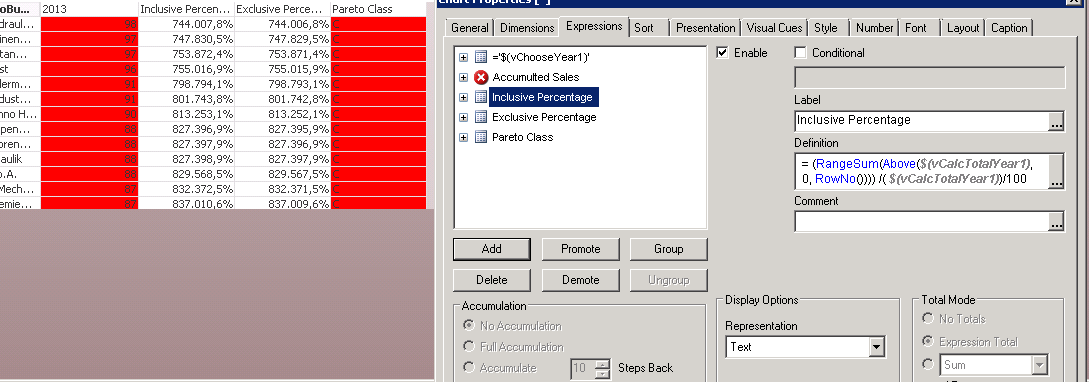I think there is again a mistake in my formula:

RangeSum(Above(\$(vCalcTotalYear1), 0, RowNo()))) /( \$(vCalcTotalYear1))/100

Or should I just try to format the second axis for the combo chart? In this case I´m not sure how to do this.

My other formulas look like this:

Sales:

\$(=vCalcTotalYear1)

Accumulted Sales

RangeSum(Above(\$(vCalcTotalYear1), 0, RowNo()))

Inclusive Percentage

RangeSum(Above(\$(vCalcTotalYear1), 0, RowNo()))) /( \$(vCalcTotalYear1))/100

Exclusive Percentage

RangeSum(Above(\$(vCalcTotalYear1), 1, RowNo()))) /( \$(vCalcTotalYear1))/100

Pareto Class

If([Exclusive Percentage] <= 0.8, 'A', If([Exclusive Percentage] <= 0.9, 'B', 'C'))

If I format the table as pivot then accumulated Sales is correct. If I format it as Straight table then it´s incorrent.  Straight Table: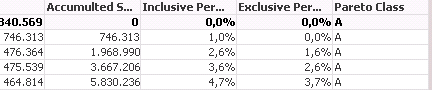236 ViewsEmployee

You cannot have the same variable in the numerator as in the denominator.

Numerator should have Sum(Sales)

Denominator should have Sum(total Sales)

Further, you do not need to divide by 100. Just format it as percentage and it will automatically display it correctly.

HIC

236 ViewsNot applicable

Hi Henric,

Thanks for such a detailed solution. Everything works fine for me except the reference line. Firstly I want to understand why we are using secondary Y for reference line. Reference line comes up nicely using Primary Y but does not show up using the secondary Y . Please help.

Thanks

Abhishek

236 ViewsEmployee

It depends what you have as primary and secondary Y. In my example, the primary Y is Sum(Sales) which is typically 30000\$, and the secondary is the relative percentage, i.e. a number between 0 and 1.

Primary and secondary are defined through the Left and Right Axis on the Axes tab.

HIC

236 ViewsNot applicable

I missed the right hand axis part for second dimension. Now I think I got it wright. Thanks for the quick help Henric. The reference line is also working for secondary Y now.

I have another question. Can we show the products in x- axis as percentage?

I tried with a calculated dimension like   "RowNo()/Count(Products)" .

I want to achieve this so that we can show clearly "First 20% products are contributing to 80% of the Sales"

236 ViewsEmployee

You could use

Count(distinct Product)/Count(distinct total Product)

as expression to calculate such a percentage.

But if you want a calculated dimension, I am not so sure what it would be...

HIC

236 ViewsPartner

Hi Henric,

Thanks for this excellent post.

Also, I was showing the Accumulated Sales % Now I want to show two Referal Lines (black dotted line): one for Top 5 and other for Top 10. How can I show it dynamically?

Here is the image below: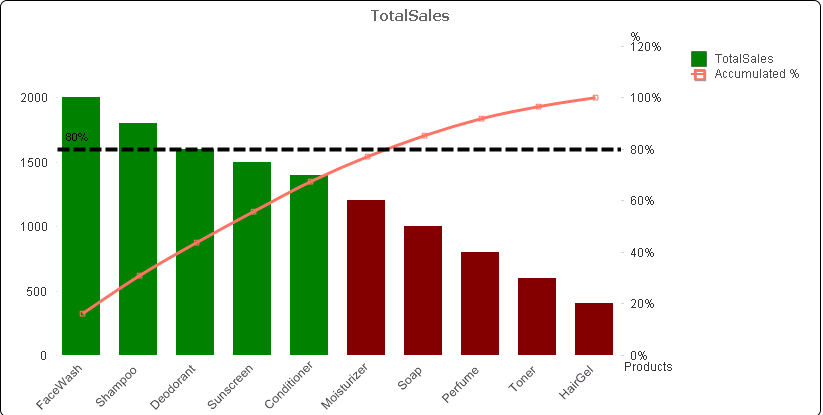236 Views
New Contributor III

I find also useful add an expression RowNo() with Text on Axis as Display Optionso you can easily know how many products make the 80% of revenue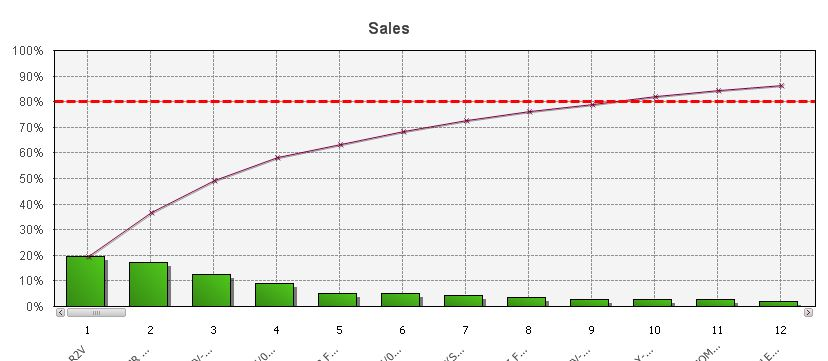236 ViewsEmployee

I would create a reference line for the top 5 using

Sum(Aggr(If(Rank(Sum (Sales))<=5,Sum (Sales)),ProductName)) / Sum (Sales)

HIC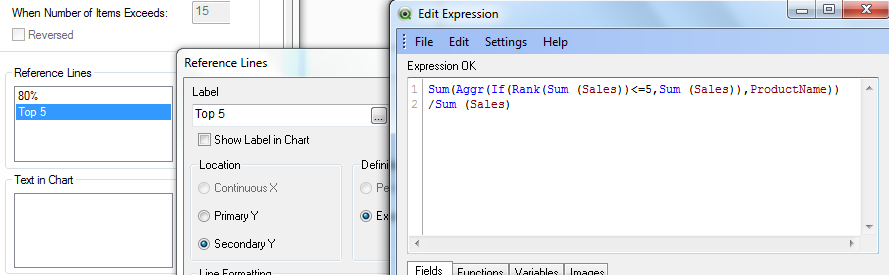236 ViewsPartner

Great Henric. It really helped in acheiving my requirement.

Learnt a new thing from this as well. Thank you very much for this!

236 ViewsNot applicable

Great post as always. I like the use of a background colour for each Pareto class.

236 ViewsNot applicable

is there any way to count number of elements in A-class and number of elements in b-class ? and if i want to put this formula in a textbox ?  thanks

236 Views
Contributor

Could this work If ([Exclusive Percentage] <= 100,count(Row)) ?

I made a count in the script instead, that seems to be more correct to use as sum of xxx as a expression.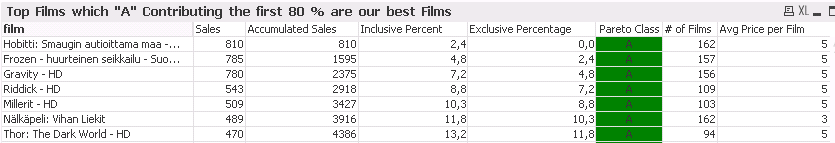/Tormod

236 ViewsNot applicable

Very useful and I have been using this already. However how does this work in a straight table or a pivot with more dimensions? The percentages will go wrong when I try this. Basically I want more dimensions for more detailed overview of the products (example including brands)

236 Views
New Contributor III

Thank you, very useful explanation!

236 ViewsNot applicable

Has anyone a idea for a pivot chart with more than one dimension? I try to represent an ABC analysis with ABC as the first dimension and for example the goods as the second dimension.

236 ViewsNot applicable

In the Running Total for Accumulated Sales, the total is adding the absolute value of the sales. I have some credits where there would be line items that are negative. Is there a way to exclude the negative values or have them properly calculated in the running total so they are not treated as an absolute value?

236 ViewsNot applicable

What`s about using the fabs expression?

236 ViewsNot applicable

This is just what I needed. Thank you!

236 ViewsNot applicable

Please advise me how to get and display the totals separately for each Pareto class?

236 Views